# ISEE Middle Level Math : Data Analysis

## Example Questions

### Example Question #31 : Data Analysis And Probability

Find the range in this set of numbers: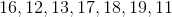Possible Answers:Correct answer:Explanation:

First, order the numbers from least to greatest: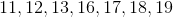Then, subtract the smallest number from the largest: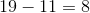Answer: The range is.

### Example Question #32 : Data Analysis And Probability

Find the range in this set of numbers: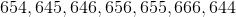Possible Answers: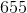Correct answer:Explanation:

First, order the numbers from least to greatest: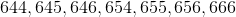Then, subtract the smallest number from the largest: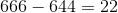### Example Question #31 : Data Analysis And Probability

Find the range of this set of numbers: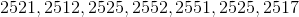Possible Answers: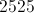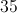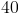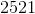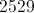Correct answer:Explanation:

First, order the numbers in the set from least to greatest: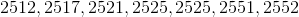Then, find the difference between the largest and the smallest numbers in the set: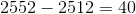Therefore, the range is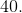### Example Question #31 : Data Analysis

Find the range of this set of numbers: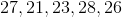Possible Answers:Correct answer:Explanation:

First, order the numbers from least to greatest: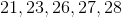Then, subtract the smallest number from the largest number: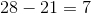Answer: The range is.

### Example Question #33 : Data Analysis

Find the range for this set of numbers: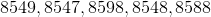Possible Answers: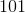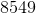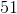Correct answer:Explanation:

First, order the numbers from least to greatest: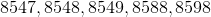Then, subtract the smallest number from the largest: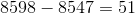Answer: The range is### Example Question #31 : Range

Find the range for this set of numbers: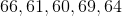Possible Answers: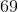Correct answer:Explanation:

First, order the numbers from least to greatest: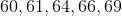Then, subtract the smallest number from the largest: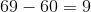Answer: The range is.

### Example Question #35 : Data Analysis

Find the range for this set of numbers: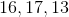Possible Answers:Correct answer:Explanation:

First order the numbers from least to greatest: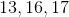Then, subtract the smallest number from the largest number: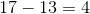Answer: The range is.

### Example Question #1 : Range

What is the range of the following data set?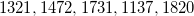Possible Answers: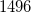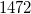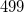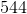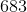Correct answer:Explanation:

The range of a data set is equal to the difference between the largest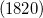and the smallest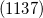numbers.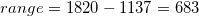### Example Question #342 : Isee Middle Level (Grades 7 8) Mathematics Achievement

Find the range in this set of numbers: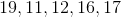Possible Answers:Correct answer:Explanation:

First, order the numbers from least to greatest: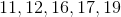Then, subtract the smallest number from the largest:Answer: The range is.

### Example Question #39 : Data Analysis And Probability

Find the range in this set of numbers: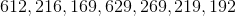Possible Answers: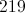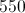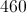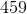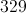Correct answer:Explanation:

First, order the numbers in the set from least to greatest: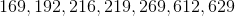Then, find the difference btween the largest and smallest numbers: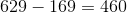Answer: The range is 460.

### All ISEE Middle Level Math Resources